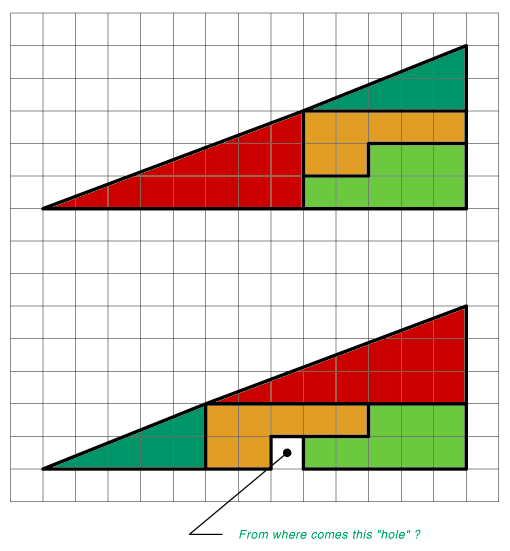# Two triangles with identical part shows difference, how is it possible?Both triangles are made of identical parts. In the bottom one is one empty square though. How is it possible?

There is a little trick – what looks like a triangle is not quite triangle in fact.
In the top image the hypotenuse is bowed inside and in the bottom image it’s bowed outside.
If you count the squares and find out how long the legs of red and green triangles are you see that their ratios are not the same which means that their angles are not the same and their hypotenuses in the picture are under a slightly different angle.

As the first answer from neha points out, it is not a triangle and you can determine that by looking at the ratios of the red and green right triangles.  The green triangle has legs of 2 and 5 (2/5 = 0.4 ratio) and the red triangle has legs of 3 and 8 (3/8 = .375 ratio).

The full right triangle has legs of 5 and 13 (5/13 = .3846…) which is between the ratios of the other two triangles.  This triangle should have an area of 5 * 13 / 2 = 32.5 squares.  Adding up the areas of the pieces we have:

Yellow: 7
Light green: 8
Green triangle: 2 * 5 / 2 = 5
Red triangle: 3 * 8 / 2 = 12

Total = 7 + 8 + 5 + 12 = 32

There is 0.5 squares missing in the top figure due to the hypotenuse being bowed in and 0.5 squares extra in the bottom figure due to the hypotenuse being bowed out.  The seemingly missing square in the bottom figure is accounted for by the 0.5 + 0.5 = 1 square.